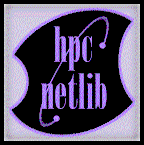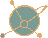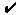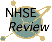High Performance Math Software

Catalog
HPC-Netlib Software CatalogPart of the National HPCC Software Exchange (NHSE)Matrix of Freely Available Linear Algebra Software from Dr. Jack Dongarra at the University of Tennessee.

Apreceding a title indicates that the software has been granted the "Checked" review level.preceding an item indicates that the software has been reviewed in an NHSE Review article.
The individual catalog entries have links to the review information.
See the explanation of review procedures. Search the Catalog
This query tool searches full-text and supports Boolean operators.

Numerical Programs and Routines
PETSc portable, extensible toolkit for scientific computation
Differential and Integral Equations
Integral equations
Ordinary differential equations
Partial differential equations
//ELLPACK problem solving environment for PDE based applications
BNALib A BASIC Numerical Analysis Library
DAGH - Distributed Adaptive Grid Hierarchy provides the framework to solve systems of partial differential equations using adaptive finite difference methods.
Diffpack the ojbect-oriented toolkit for numerical solution of PDEs
DOUG - Domain decomposition On Unstructured Grids a black box parallel iterative solver for finite element systems arising from elliptic partial differential equations.
MODULEF finite element library
MUDPACK a collection of vectorized portable Fortran 77/90 subprograms which efficiently solve linear elliptic Partial Differential Equations (PDEs) using multigrid iteration
Unstructured Grids (UG) for the adaptive multigrid solution of partial differential equations
VECFEM - VECtorized Finite Element Method for solution of nonlinear PDEs
Graph and mesh partitioning
CHACO software for partitioning and ordering graphs
GEOMPACK for generation of finite element meshes using geometric algorithms
GRUMMP (Generation and Refinement of Unstructured Mixed-Element Meshes in Parallel) automatic mesh generation software for unstructured meshes with mixed element types
JOSTLE mesh partitioning software
METIS unstructured graph partitioning and sparse matrix ordering system
ParMETIS A Parallel Graph Partitioning and Sparse Matrix Ordering Library
PARTY graph partitioning library
PMHS - 3D Parallel Multigrid Helmholtz Solver See Abstract
PPGP A Parallel Partitioner for Unstructured Grids and Random Particles
SCOTCH Static Mapping and Graph Partitioning Package for mapping a weighted source process graph onto a weighted target architecture graph
Triangle a 2D finite element mesh generator and Delaunay triangulator
Linear algebra
ATLAS (Automatically Tuned Linear Algrebra Software) is an approach for the automatic generation and optimization of numerical software for processors with deep memory hierarchies and pipelined functional units.Aztec a parallel iterative library for solving sparse linear systems
BLACS Basic Linear Algebra Communication Subprograms
BLAS (Basic Linear Algebra Subroutines) building blocks for constructing efficient and portable linear algebra software for high-performance computers.
Blitz++ Scientific Computing in C++
BlockSolve for solution of large, sparse linear systems on massively parallel computers
BNALib A BASIC Numerical Analysis Library
CAPSS sparse direct linear systems software
Complex Dense Linear Solver None Available
Elegant Mathematics Iterative Solvers preconditioners and iterative methods for solving very large linear systems
f2j Fortran to Java converter
GAMS D1b6. Matrix Multiplication scalable implementation of common matrix multiplication operations
General Purpose Solver (GPS) high performance solvers for linear systems of equations that arise from structural engineering, electromagnetics, and other scientific and engineering applications
GMRES for real and complex, single and double precision arithmetics suitable for serial, shared memory and distributed memory computers
IML++ (Iterative Methods Library) a C++ templated library of modern iterative methods for solving linear systems of equations
Intel Performance Library Suite a set of libraries optimized for Intel processors addressing linear algebra, signal processing, and speech and character recognition requirements.
LAPACK is a library of numerical linear algebra subroutines designed for high performance on workstations, vector computers, and shared memory multiprocessors
LASPack for solving large sparse systems of linear equations such as those arising from discretization of partial differential equations
Matrix Template Library (MTL) is a high-performance generic component library that provides comprehensive linear algebra functionality for a wide variety of matrix formats
MP_SOLVE is used to solve sparse, irregular systems of equations with multiple right-hand sides on distributed memory, multiprocessor computers having the MPI communications library
NIST Sparse BLAS computational kernels for fundamental sparse matrix operations
P-SPARSLIB a portable library of distributed memory sparse iterative solversParallel Iterative Methods (PIM) Package a collection of Fortran 77 routines designed to solve systems of linear equations (SLEs) on parallel computers using a variety of iterative methods.
PARPACK for solving large scale eigenvalue problems on distributed memory parallel architectures
PARPRE parallel preconditioners for large sparse iterative solversPCG - parallel Preconditioned Conjugate Gradient package for solving sparse linear systems by iterative methods on scalar, vector, and parallel computers
PETSc portable, extensible toolkit for scientific computation
PLAPACK - Parallel Linear Algebra Package MPI based package for building dense linear algebra libraries
PSPASES (Parallel Sparse Symmetric Direct Solver Library) scalable parallel direct solver library for sparse symmetric positive definite linear systems
Scalable Basic Linear Algebra Subprograms (sB_BLAS) scalable implementation of common matrix-matrix operations
ScaLAPACK dense linear algebra routines for distributed memory computers
SPARSE a set of C procedures for solving large sparse real or complex linear systems.SparseLib++ class library for efficient sparse matrix computations across various computational platforms
SparsLinC to support the computation of linear combinations of sparse vectors for single and double precision real and complex types.
SPLIB library of sparse iterative solvers, with preconditioners, for rapid prototyping of solvers for nonsymmetric linear systems of equations
Spooles for solving sparse linear systems of equations
SuperLU_MT parallel sparse Gaussian Elimination for shared memory machines
SYmmetric Invariant Subspace Decomposition Approach (SYISDA) eigensolver package or finding all eigenvalues and eigenvectors of real symmetric diagonalizable matrices in parallel
Templates for the Solution of Linear Systems: Building Blocks for Iterative Methods a description of a general algorithm rather than the executable object code or the source code more commonly found in a conventional software library.
UMFPACK Unsymmetric-pattern Multifrontal Package
Miscellaneous
3DFFT To provide Fortran routines for performing three-dimensional Fast Fourier Transforms in parallel on multiple processors.
ADIC a tool for the automatic differentiation of programs written in ANSI C.
ADIFOR automatic differentiation of Fortran codes
ExacMath is a math library that supports real and complex arithmetic with three floating point data types.
FFTW fast, free C FFT library including real, multidimensional, and parallel transforms
Parallel Genetic Algorithm Library (PGAPack) a general-purpose, data-structure-neutral, parallel genetic algorithm library.
Parallel Software for Implicit Differential Equations (PSIDE) is a Fortran 77 code for solving implicit differential equations on shared memory parallel computers.
Parallel Sorting by Regular Sampling (PSRS) three implementations of a parallel sort using the algorithm of Parallel Sorting by Regular Sampling
ParSODES a parallel stiff ODE solver
PseudoPack for numerical differentiation by pseudospectral methods
RAPID integrates automatic scheduling techniques and efficient communication schemes for irregular task computations with mixed granularities on message-passing distributed memory machines.
SPRNG a set of libraries for scalable and portable pseudorandom number generation
V_SQRT A Library Routine for Computing Square Roots of Double-Precision Vectors on CRAY
Optimization
BNALib A BASIC Numerical Analysis Library
COOOL -- CWP Object Oriented Optimization Library collection of C++ class libraries for handling mathematical optimization problems
CUTE - Constrained and Unconstrained Testing Environment The Constrained and Unconstrained Testing Environment (CUTE) is a suite of Fortran subroutines, scripts and test problems for linear and nonlinear optimization.
MINPACK-2 optimization software for high performance computers
PDS direct search methods for unconstrained optimization
This catalog was generated Thr Jul 8 16:43:30 1999 using Repository In a Box (RIB) version 1.2
from local Assets